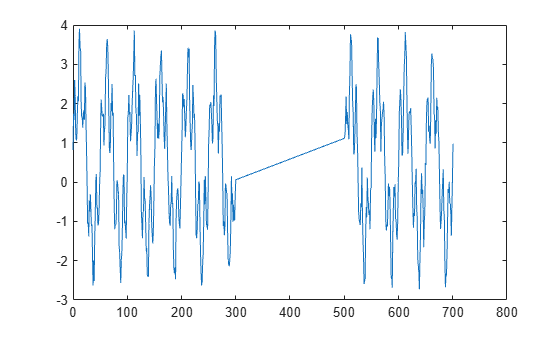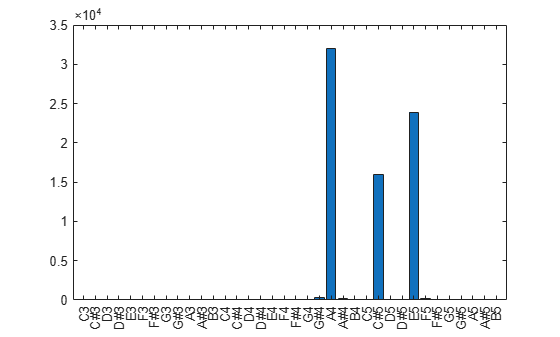# nufft

Nonuniform fast Fourier transform

## Syntax

``Y = nufft(X,t)``
``Y = nufft(X,t,f)``
``Y = nufft(X,t,f,dim)``
``Y = nufft(X)``

## Description

example

````Y = nufft(X,t)` returns the nonuniform discrete Fourier transform (NUDFT) of `X` using the sample points `t`. If `X` is a vector, then `nufft` returns the transform of the vector.If `X` is a matrix, then `nufft` treats the columns of `X` as vectors and returns the transform of each column.If `X` is a multidimensional array, then `nufft` treats the values along the first array dimension whose size does not equal 1 as vectors and returns the transform of each vector. ```

example

````Y = nufft(X,t,f)` computes the NUDFT at the query points `f` using the sample points `t`. To specify `f` without specifying sample points, use `nufft(X,[],f)`.```
````Y = nufft(X,t,f,dim)` returns the NUDFT along dimension `dim`. For example, `nufft(X,t,f,2)` computes the transform of each row of a matrix `X`.```
````Y = nufft(X)` returns the discrete Fourier transform of `X`, and is equivalent to `fft(X)`.```

## Examples

collapse all

Create a signal `X` sampled at unevenly spaced points `t`. Compute the nonuniform fast Fourier transform `Y`.

```t = [0:300 500.5:700.5]; S = 2*sin(0.1*pi*t) + sin(0.02*pi*t); X = S + rand(size(t)); Y = nufft(X,t);```

Plot the absolute value of the transform as a function of the default frequencies.

```n = length(t); f = (0:n-1)/n; plot(f,abs(Y))```Define and label the frequencies of a range of musical tones.

```C3 = 440 / (2^(21/12)); nOctaves = 3; musicalTones = C3 * 2.^((0:(12*nOctaves-1))/12); toneNames = ["C";"C#";"D";"D#";"E";"F";"F#";"G";"G#";"A";"A#";"B"] + string(3:(3+nOctaves-1)); toneNames = categorical(toneNames, toneNames);```

Define the audio signal sampling frequency in Hz, the sample points `n`, and a signal containing a major chord `X`.

```fs = 16e3; n = 1:16000; X = 4*cos(2*pi*(440/fs)*n) + 2*cos(2*pi*(554.37/fs)*n) + 3*cos(2*pi*(659.2/fs)*n);```

Compute and plot the frequency components of the major chord.

```Y = nufft(X,[],musicalTones/fs); bar(toneNames(:),abs(Y))```## Input Arguments

collapse all

Input array, specified as a vector, matrix, or multidimensional array.

Data Types: `double` | `single` | `int8` | `int16` | `int32` | `uint8` | `uint16` | `uint32` | `logical`
Complex Number Support: Yes

Sample points, specified as a vector of length n, where n is the length of the operating dimension of the input array `X`. By default, the sample points vector is `0:(n-1)`.

Data Types: `double` | `single`

Query points, specified as a vector. By default, the query points vector is `(0:(n-1))/n`, where n is the length of the operating dimension of the input array `X`. To specify `f` without specifying sample points, use `nufft(X,[],f)`.

Data Types: `double` | `single`

Dimension to operate along, specified as a positive integer scalar. The default is the first array dimension whose size does not equal 1.

Data Types: `double` | `single` | `int8` | `int16` | `int32` | `int64` | `uint8` | `uint16` | `uint32` | `uint64` | `logical`

collapse all

### Nonuniform Discrete Fourier Transform of Vector

For a vector X of length n, sample points t, and frequencies f, the nonuniform discrete Fourier transform of X is defined as

`$Y\left(k\right)=\sum _{j=1}^{n}X\left(j\right){e}^{-2\pi i\text{\hspace{0.17em}}t\left(j\right)\text{\hspace{0.17em}}f\left(k\right)}$`

where k = 1, 2, …, m. When t = 0, 1, …, n-1 and f = (0, 1, …, n-1)/n (defaults for `nufft`), the formula is equivalent to the uniform discrete Fourier transform used by the `fft` function.

 Potter, Samuel F., Nail A. Gumerov, and Ramani Duraiswami. “Fast Interpolation of Bandlimited Functions.” In 2017 IEEE International Conference on Acoustics, Speech and Signal Processing (ICASSP), 4516–20. New Orleans, LA: IEEE, 2017. https://doi.org/10.1109/ICASSP.2017.7953011.

 Dutt, A., and V. Rokhlin. “Fast Fourier Transforms for Nonequispaced Data.” SIAM Journal on Scientific Computing 14, no. 6 (November 1993): 1368–93. https://doi.org/10.1137/0914081.